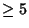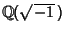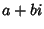## Algebraic Integer

Ifis a Root of the Polynomial equationwhere thes are Integers andsatisfies no similar equation of degree, thenis an algebraic Integer of degree. An algebraic Integer is a special case of an Algebraic Number, for which the leading Coefficientneed not equal 1. Radical Integers are a subring of the Algebraic Integers.

A Sum or Product of algebraic integers is again an algebraic integer. However, Abel's Impossibility Theorem shows that there are algebraic integers of degreewhich are not expressible in terms of Addition, Subtraction, Multiplication, Division, and the extraction of Roots on Real Numbers.

The Gaussian Integers are algebraic integers of, sinceare roots ofReferences

Hancock, H. Foundations of the Theory of Algebraic Numbers, Vol. 1: Introduction to the General Theory. New York: Macmillan, 1931.

Hancock, H. Foundations of the Theory of Algebraic Numbers, Vol. 2: The General Theory. New York: Macmillan, 1932.

Pohst, M. and Zassenhaus, H. Algorithmic Algebraic Number Theory. Cambridge, England: Cambridge University Press, 1989.

Wagon, S. Algebraic Numbers.'' §10.5 in Mathematica in Action. New York: W. H. Freeman, pp. 347-353, 1991.